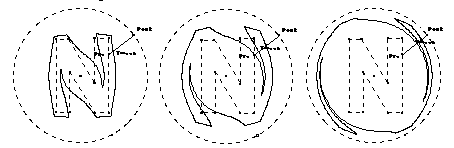Home > General Resources > Recent Talks > Computer Graphics > Ideas, Problems, and Investigations

# Ideas, Problems, and Investigations in Computer Graphics

The following annotated sketches explore various ideas and problems drawn from computer graphics applications in all of these areas.

### Studies in Shape

#### Morphing Letterforms

If a single geometric transformation has captured the imagination of the special effects industry in the 1990s, it's "morphing." When morphing, an arbitrary shape transforms dynamically to take on the appearance of some other shape. At the movies, science fiction and horror genres have disported the morphing process in no end of screenplays rich with shape-shifters, werewolves, and other metamorphic agents (for example, John Carpenter's The Abyss or James Cameron's Terminator II). Conventional human forms emerge dramatically from shapeless blobs of ectoplasm: no mathematician would call morphing a rigid transformation!

A simplified form of morphing can be explored in planar geometry by transforming one (arbitrary) simple polygon into another. In overview, the process involves identifying a mapping between all points on the perimeter of the first shape (the pre-image) and points on the perimeter of the second (the image). Once such a mapping has been defined, morphing the pre-image into the image simply involves sending pre-image points (representing the pre-image's perimeter) to their corresponding image points so that they arrive at their destination simultaneously.

The problems to solve in constructing a morphing of shapes are to identify mathematically both the mapping of pre-image to image points, and to specify the mechanism for moving the former to the latter.Figure 1. Mapping Pre to Post

One solution to these problems is approached by Figure 1, which considers the morph of a letterform N (represented by an irregular 10-gon) and the letterform O (represented by a circle). A mapping between the two shapes has been defined by first placing the pre-image (N) completely within the image (O), and then drawing a ray from the center of O through some point Pre on the pre-image. The intersection of the ray with the image circle is defined as point Post, and since any ray from the center of a circle must intersect that circle, this construction guarantees that every point Pre on the pre-image corresponds to some other point Post on the image.

It's worth momentarily considering this mapping as a mathematical function, which for each input value Pre, generates an output value Post, or Post=f(Pre). The domain of the function is N; the range O. Is the function one-to-one? Inspection of Figure 1 suggests not: the mapping ray passes through three points on the perimeter of the pre-image (three possible values of Pre) all of which correspond to the same point Post.

Once the mapping has been established, generating intermediate images of the morph is straightforward. Consider the segment between Pre and Post. This segment determines the domain of each point on the perimeter of the morphing polygon, as it evolves from N to O. Thus the midpoint of this segment represents a point on the perimeter of a polygon morphed "half-way" between pre-image and image. In general, any point Tween on this segment corresponds to a point on the perimeter of an intermediate morph we can call the mid-image.

What does the mid-image look like for a given position of Tween? Clearly, if Tween and Pre are coincident, then the pre-image and the mid-image are identical: they form an N. If Tween and Post are coincident, then the mid-image is the same as the image: O. Intermediate values of Tween describe mid-images that are |PreTween|/|PrePost| of the way between N and O.

In Sketchpad, the mid-image corresponding to a given point Tween (on the segment between Pre and Post) can be generated by constructing the locus of Tween as Pre travels about the pre-image. If Tween is an arbitrary point on the segment between Pre and Post (as opposed to some specific point, such as the midpoint), then it can be dragged freely between the segment's endpoints. Correspondingly, the locus of Tween morphs smoothly between N and O, as illustrated in Figure 2.Figure .: Morphing N to O

Defining morphed images as the locus of Tween is a geometric application of linear interpolation: Tween is interpolated on the (linear) segment between Pre and Post. But morphing can be a more compelling application of linear interpolation than the traditional mode by which it's introduced to students, where it's used to find values not explicitly mentioned in the log tables at the back of a textbook.

#### Further Exploration

Students who have covered the basics of morphing can go on to investigate a number of more detailed questions.

• This example morphs between a polygon and a circle. How might one construct the morph between a polygon and a line (or a segment)? Or between one arbitrary polygon and another?
• Imagine if Tween were defined on the line passing through Pre and Post, rather than on the segment between them. What would happen to the morphed mid-image if Tween was no longer between Pre and Post? (This is extrapolation, as opposed to interpolation.)
• How would you define a morphing mapping between one photograph and another, where you were concerned not just with the perimeter of shapes but with their interior details as well?

 For accuracy's sake, I should note that the term morphing is usually reserved for transitions between fully colored and shaded pre-images and images. The process of morphing only the perimeter of shapes is known in the computer graphics community as tweening, and was used in the early days of computer graphics to generate automatic, intermediate movie frames between "key-frames" that were hand-drawn by a professional illustrator in animated pictures (cartoons). The tweened frames were then colored by hand, and if the number of key frames was sufficiently high, the audience tended not to notice that animated characters were moving not according to the laws of cartoon anatomy but according to strict linear interpolation.